Courses

# Retro (Past 13 Year) IIT- JEE Advanced (Chemical Kinetics)

## 10 Questions MCQ Test Chemistry for JEE Advanced | Retro (Past 13 Year) IIT- JEE Advanced (Chemical Kinetics)

Description
This mock test of Retro (Past 13 Year) IIT- JEE Advanced (Chemical Kinetics) for JEE helps you for every JEE entrance exam. This contains 10 Multiple Choice Questions for JEE Retro (Past 13 Year) IIT- JEE Advanced (Chemical Kinetics) (mcq) to study with solutions a complete question bank. The solved questions answers in this Retro (Past 13 Year) IIT- JEE Advanced (Chemical Kinetics) quiz give you a good mix of easy questions and tough questions. JEE students definitely take this Retro (Past 13 Year) IIT- JEE Advanced (Chemical Kinetics) exercise for a better result in the exam. You can find other Retro (Past 13 Year) IIT- JEE Advanced (Chemical Kinetics) extra questions, long questions & short questions for JEE on EduRev as well by searching above.
QUESTION: 1

### Only One Option Correct Type This section contains 10 multiple choice questions. Each question has four choices (a), (b), (c) and (d), out of which ONLY ONE is correct. Q.  For the elementary reaction M → N, the rate of disappearance of M increases by a factor of 8 upon doubling the concentration of M. The order of the reaction with respect to M is (2014 Adv., Only One Option Correct Type)

Solution:

For the elementary reaction, M → N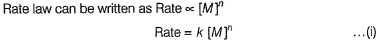rate becomes 8 times, hence new rate law can be written as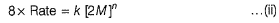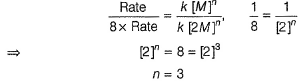QUESTION: 2

### In the reaction ,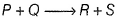the time taken for 75% reaction of P is twice the time taken for 50% reaction of P. The concentration of Q varies with reaction time as shown in the figure . The over all order of the reaction is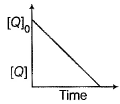(2013 Adv., Only One Option Correct Type)

Solution:

Plan Time of 75% reaction is twice the time taken for 50% reaction if it is first order reaction w.r.t P. From graph, [Q] decreases linearly with time, thus it is zeroth order reaction w.r.t. Q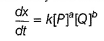Order w.r.t. P = a = 1
Order w.r.t. Q = b = 0
Thus, overall order of the reaction = 1 + 0 = 1

*Answer can only contain numeric values
QUESTION: 3

### An organic compound undergoes first order decomposition . The time taken for its decomposition to 1/8 and 1/10 of its initial concentration are t 1/8 and t 1/10 respectively. What is the value of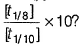(log10 2 = 0.3) (2012, Integer Type)

Solution:

For a first order process,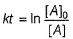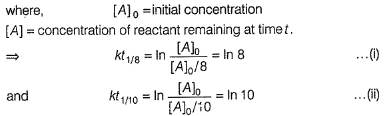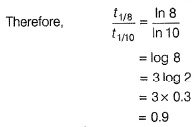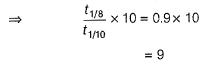*Multiple options can be correct
QUESTION: 4

For the first order reaction,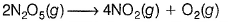(2011, One or More than One Options Correct Type)

Solution:

(a) For a first order reaction, the concentration of reactant remaining after time t is given by [A] = [A]0 e-kt

Therefore, concentration of reactant decreases exponentially with time.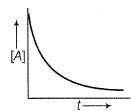(b) Rise in temperature increases rate constant (k) and therefore decreases half-life (t1/2) as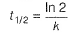(d) For a first order reaction, if 100 moles of reactant is taken initially, after n half-lives, reactant remaining is given by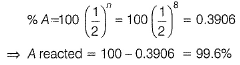(c) Half-life of first order reaction is independent of initial concentration.

QUESTION: 5

Plots showing the variation of the rate constant (k) with temperature (T) are given below. The plot that follows Arrhenius equation is

(2010, Only One Option Correct Type)

Solution:

According to Arrhenius equation, rate constant increases exponentially with temperature:
k = Ae -Ea/RT

*Answer can only contain numeric values
QUESTION: 6

The concentration of R in the reaction R → P was measured as a function of time and the following data is obtained :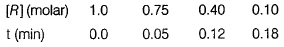Q.

The order of the reaction is

(2010 , inte ger Type)

Solution: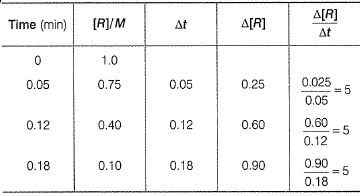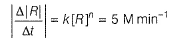Rate remains constant throughout thus, order = zero

QUESTION: 7

For a first order reaction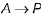, the temperature (T) dependent rate constant (k) was found to follow the equation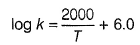The pre-exponential factor A and the activation energy Ea, respectively, are

(2009, Only One Option Correct Type)

Solution:

The logarithmic form of Arrhenius equation is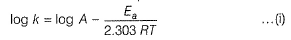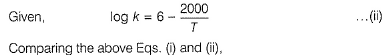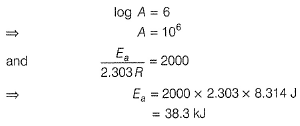QUESTION: 8

Under the same reaction conditions, in itial concentration of 1.386 mol dm -3 of a substance becomes half in 40 s and 20 s through first order and zero order kinetics respectively. Ratio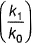of the rate constants for first order (k1) and zero order (k0) of the reaction is

(2008, Only One Option Correct Type)

Solution:

For first order reaction,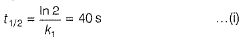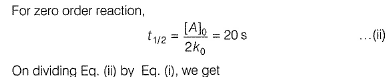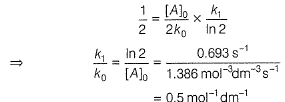QUESTION: 9

Consider a reaction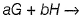products. When concentration of both the reactants G and H is doubled, the rate increases by eight times . Flowever, when concentration of G is doubled keeping the concentration of H fixed, the rate is doubled. The overall order of the reaction is

(2007, Only One Option Correct Type)

Solution: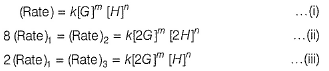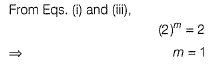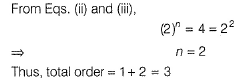QUESTION: 10

Which one of the following statements is incorrect about order of reaction?

(2005, Only One Option Correct Type)

Solution:

Order of a reaction can take any real value, i.e. negative, integer, fraction, etc.